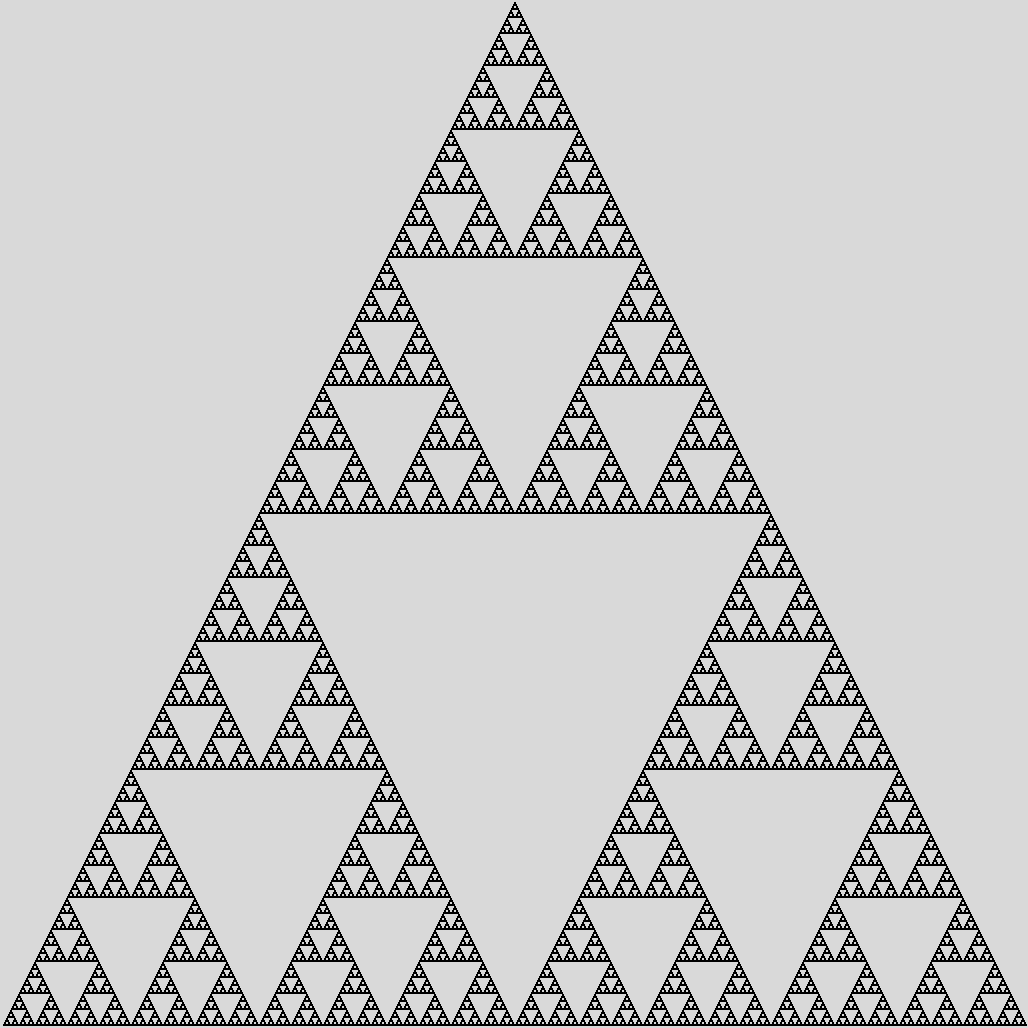# Week 3 notes

Comp 163-002, Spring 2022, MW 4:15-5:30, Mondays in Cuneo 117 (starting January 31) and Wednesdays online.

The primary goal of this course is to become familiar with some of the basic mathematical ideas used in programming.

Levin, 1.1, 1.2

Counting: Levin 1.1, on page 57

• Multiplicative principle
• Set-theoretic approach
• Pies on page 64

Counting subsets of {1, 2, ..., N}, Levin 1.2 on page 70

• Counting all subsets (including ∅)
• Counting all nonempty subsets
• Counting all subsets of size 1
• Counting all subsets of size 3; = # of subsets of size 2
• Binomial coefficients
• Bit Strings, B(n,k) = # of bit strings of length n with k 1's (weight k)
• Recurrence relation: B(5,3) = B(4,3) + B(4,2)
• Lattice Paths

Wednesday

Probably the best way to understand counting all k-element subsets of {1,2,...,n} is first to count the k-element sequences (with no repeats),
using the multiplication principle, and then divide by k! because one set of size k corresponds to k! sequences.

Levin does this in Section 1.3.

Recurrence relationships:

factorial: fact(1) = 1, fact(n+1) = (n+1)*fact(n)

fib(0) = fib(1) = 1, fib(n+1) = fib(n) + fib(n-1)

Now for C(n,k), or (n choose k). Let us define it to be n! / (n-k)!k!, or n*(n-1)*...*(n-k+1) / k!.

Claim: C(n,0) = C(n,n) = 1, C(n+1,k) = C(n,k) + C(n,k-1)

Claim: number of k-weight bitstrings of length n is equal to the number of k-sized subsets of {1,...,n}.

Proof: Natural one-to-one correspondence of bitstring s to {i<=n | s(i) = 1}, that is,  <0,1,1,0,0,0,1,0> matches to {2,3,7}
(wait, are bitstrings indexed starting at 0 or starting at 1?)

Recurrence relation for bitstrings: B(n,0) = B(n,n) = 1, B(n+1,k) = B(n,k) + B(n,k-1)

Therefore B(n,k) = C(n,k)

This is an alternative proof!

Pascal's triangle, using the recurrence rule

Binomial theorem: expansion of (x+y)n

Counting lattice paths: same as bitstrings, where 1 = horizontal and 0 = vertical

Pascal's Triangle

1
1   1
1   2   1
1   3   3   1
1   4   6   4   1
1   5  10  10   5   1
1   6  15  20  15   6   1
1   7  21  35  35  21   7   1
1   8  28  56  70  56  28   8   1

Pascal's triangle modulo 2: